# Electron Lens

(redirected from electron lenses)
Also found in: Dictionary, Thesaurus.

## Electron lens

An electric or magnetic field, or a combination thereof, which acts upon an electron beam in a manner analogous to that in which an optical lens acts upon a light beam. Electron lenses find application for the formation of sharply focused electron beams, as in cathode-ray tubes, and for the formation of electron images, as in infrared converter tubes, various types of television camera tubes, and electron microscopes.

Any electric or magnetic field which is symmetrical about an axis is capable of forming either a real or a virtual electron image of an object on the axis which either emits electrons or transmits electrons from another electron source. Hence, an axially symmetric electric or magnetic field is analogous to a spherical optical lens.

The lens action of an electric and magnetic field of appropriate symmetry can be derived from the fact that it is possible to define an index of refraction for electron paths in such fields. This index depends on the field distribution and the velocity and direction of the electrons.

Electron lenses differ from optical lenses both in that the index of refraction is continuously variable within them and in that it covers an enormous range. Furthermore, in the presence of a magnetic field, the index of refraction depends both on the position of the electron in space and on its direction of motion. It is not possible to shape electron lenses arbitrarily. See Electrostatic lens, Magnetic lens

McGraw-Hill Concise Encyclopedia of Physics. © 2002 by The McGraw-Hill Companies, Inc.
The following article is from The Great Soviet Encyclopedia (1979). It might be outdated or ideologically biased.

## Electron Lens

a device designed to shape and focus electron beams and to use such beams to produce electron-optical images of objects and parts of objects. Devices used to perform such operations on ion beams are called ion lenses. The action on the electron or ion beam is exerted by an electric or magnetic field, and the lenses are called electrostatic or magnetic lenses, respectively.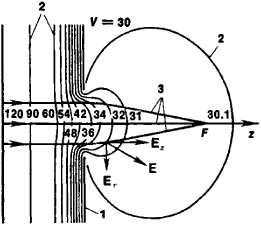Figure 1. Diaphragm with circular aperture (converging diaphragm): (1) electrode-diaphragm, (2) intersections of equipotential surfaces of the electrostatic field and the plane of the figure, (3) electron trajectories, (F) focal point of the lens. A uniform field borders the diaphragm on the left. Corresponding potential values are shown in arbitrary units next to the equipotential lines. It is assumed that the potential is equal to zero wherever the particle velocity is equal to zero. V = 30 is the potential of the electrode. The longitudinal component Ez, of the electric field E decelerates electrons, and the transverse component Er focuses electrons.

Electron lenses are classified according to the type of field symmetry and other characteristic features of the field. In many cases the terminology used to describe electron lenses is borrowed from the classical optics of light rays. This is due to the consistent analogy between the optics of light rays and electron and ion optics as well as to considerations of convenience and clarity of presentation.

The simplest axially symmetric electrostatic lens consists of a diaphragm with a circular aperture whose field borders uniform electric fields on one or both sides (Figure 1). Depending on the potential distribution, the lens may be a converging lens (producing a beam of charged particles) or a diverging lens. If there is no field on either side of an axially symmetric electrostatic lens (that is, if the lens is bordered by regions of space with constant potentials V1 and V2) and if the potentials are different, then the lens is called a bipotential, or immersion, electron lens (Figure 2); if the potentials are the same, then the lens is called a unipotential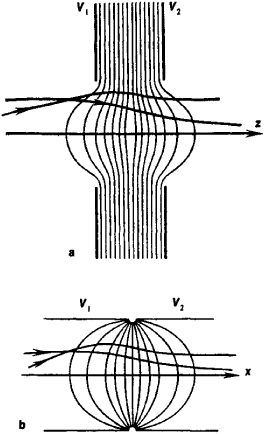Figure 2. Immersion electron lenses consisting of (a) two diaphragms and (b) two cylinders: (V1) and (V2) potentials of the electrodes. The thin lines are intersections of equipotential surfaces and the plane of the figure. The curved lines with arrows represent the trajectories of charged particles.

lens; such a lens consists of three or more electrodes. An immersion lens changes the velocity of electrons passing through it; a unipotential lens does not alter electron velocities. Both immersion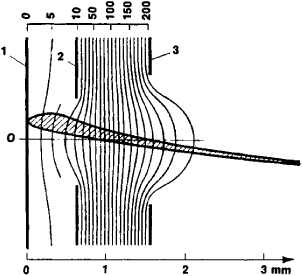Figure 3. Cathode lens: (1) cathode, (2) focusing electrode, (3) anode, (O) one of the points on the cathode that emit electrons. The thin lines represent intersections of equipotential surfaces and the plane of the figure. The values of the potentials are entered on the upper scale (the potential of the cathode is assumed to be zero). The hatched area is a cross section of the region occupied by electron flow.

and unipotential lenses always constitute converging lenses.

In some electrostatic lenses one of the electrodes is an electron-emitting cathode (cathode lenses). A lens of this type accelerates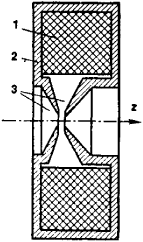Figure 4. Magnetic lens with pole pieces: (1) exciting winding, (2) casing, (3) pole pieces. The casing serves as the magnetic circuit. The pole pieces concentrate the magnetic field in a small region near the optical axis z of the system.Figure 5. Cylindrical electrostatic lenses: (a) diaphragm with slit, (b) immersion lens consisting of two pairs of plates. In the region through which charged particles pass, the fields of the lenses are unaltered in the direction parallel to the diaphragm slits or to the gaps between the plates of neighboring electrodes.

electrons emitted by the cathode to form an electron beam. A cathode lens consisting of just two electrodes—a cathode and an anode—cannot focus an electron beam, and for this purpose an additional electrode, called a focusing electrode (Figure 3), is included in the lens design.

Axially symmetric magnetic lenses are made in the form of coils of insulated wire, usually housed in an iron casing to amplify and concentrate the lens’s magnetic field. The construction of lenses with very small focal lengths requires that the extent of the field be reduced as much as possible. Pole pieces (Figure 4) are used for this purpose. The field of a magnetic lens can also be generated by a permanent magnet.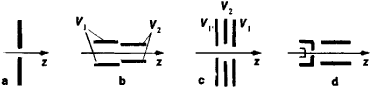Figure 6. Cross sections of electrodes of cylindrical electrostatic lenses in the plane passing through the axis z perpendicular to the midplane: (a) cylindrical-unipotential lens, (b) cylindrical immersion lens, (c) cylindrical single lens, (d) cylindrical cathode lens; (V1) and (V2) potentials of the corresponding electrodes

The electrodes of cylindrical electrostatic lenses (those exhibiting plane symmetry) are usually diaphragms with a slit or plates positioned symmetrically with respect to the midplane of the lens (Figure 5). Such lenses may be termed cylindrical, because they act on beams of charged particles just as cylindrical optical lenses act on light beams, focusing them in only one direction. The classification of cylindrical electron lenses is similar to that given for axially symmetric electron lenses; there are immersion, unipotential, cathode, and other types (Figure 6). Magnetic electron lenes, usually with an iron casing, may also be cylindrical.

The fields of transaxial electrostati lenses (Figure 7) have rotational symmetry with respect to an a is (the x-axis in the figure)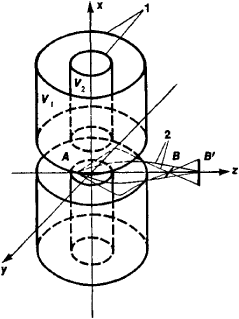Figure 7. Transaxial electrostatic lens with electrodes in the form of two coaxial tubes and with annular slits for the passage of particle beams: (1) tubular electrodes, (2) trajectories of charged particles, (V1) and (V2) potentials of the electrodes. A beam emerges from point A on the object, passes through the field of the lens, becomes astigmatic, and forms two line images B and B′. If the lens parameters are properly chosen, the lens will produce a stigmatic (point-for-point) image.

positioned perpendicular to the optical axis z of the system. In cross sections parallel to the midplane yz of such a lens, the equipotential surfaces have the form of circles or, if the field is limited, arcs, analogous to cross sections of the spherical surfaces of optical lenses. The aberrations of a transaxial lens in a direction parallel to the midplane are therefore comparable in magnitude to the aberrations of optical lenses—that is, they are very small. The line image B’ of a point or rectilinear object perpendicular to the midplane will undergo practically no aberrational expansion.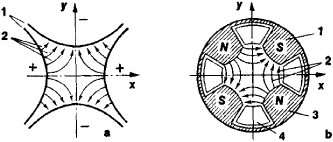Figure 8. Quadrupole electron lenses in cross sections perpendicular to the direction of motion of a beam of charged particles: (a) electrostatic lens, (b) magnetic lens; (1) electrodes, (2) lines of force, (3) pole piece, (4) exciting winding

Quadrupole electrostatic and magnetic lenses constitute a special class of electron lenses. Their fields have two planes of symmetry, and their field vectors in the region where the charged particles move are nearly perpendicular to the velocity vectors of the charged particles (Figure 8). Such lenses focus the beam in one direction and cause it to diverge in another perpendicular to the first, creating a linear image of a point object. Two quadrupole electron lenses whose fields are turned 90° to each other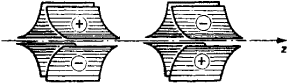Figure 9. Doublet consisting of two quadrupole electrostatic lenses whose fields are turned 90° to each other about the optical axis z of the system

about their common optical axis can be mounted one behind the other to form a doublet (Figure 9). The resulting system converges a beam in two mutually perpendicular directions and gives a stigmatic image (one in which a point is represented by a point) when the electron lens parameters are properly selected. Quadrupole electron lenses can act on beams of charged particles with much higher energies and, in the case of magnetic lenses, with larger masses than can axially symmetric electron lenses.

### REFERENCES

See references under .

V. M. KEL’MAN and I. V. RODNIKOVA